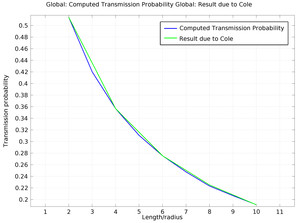# Application Gallery

#### Molecular Flow Through a Microcapillary

Application ID: 10014

Computing molecular flows in arbitrary geometries produces complex integral equations that are very difficult to compute analytically. Analytic solutions are, therefore, only available for simple geometries. One of the earliest problems solved was that of gas flow through tubes of arbitrary length, which was first treated correctly by Clausing. Later, the integral expressions he derived were computed more accurately by Cole. These authors derived values for the transmission probability of molecules incident on a tube of arbitrary length, which is independent of the pressure, provided that the Knudsen number is much greater than one (in the molecular flow regime).

This model uses the Free Molecular Flow interface to compute the transmission probability for the molecular flow of molecules through a microcapillary with different length / radius ratios. The results are compared with the accurate solution as computed by Cole.This model example illustrates applications of this type that would nominally be built using the following products: